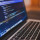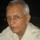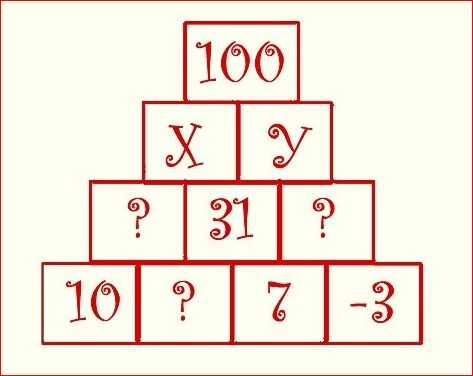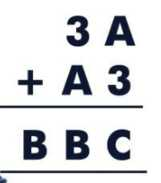Tough Puzzles & Brain Teasers
Puzzles on logic and mathematics that will make you scratch your head.
76 Members
Join this group to post and comment.Aswini Vi
Computer Science
25 Jan 2019

# Solve the below algebraic brain teaser by finding the values of A,B & C?

Find the values for A,B & C?2mos agoAswini Vi
Computer Science
2mos ago

Sorry ,for my mistake regarding the post of question incompletely @Ramani Aswath sir.

I'm again posting the question .Find the values which are  in the place of X, Y, "?"2mos ago

@Aswini Variganji , is not the above from another question?

There is no A, B, or C in the question above.Aswini Vi
Computer Science
2mos ago

The pic was attached unintentionally by mistake.

The correct question isFind A,B,&C values?2mos ago

A=7,  B=1,  C=02mos ago

A+3 gives C in the sum. Next we again have 3+A. But this gives B in the sum. This is possible only if A+C has a carryover. The carryover has to be 1, which is the maximum possible when two single digits are added. B has to be C+1.

A+3+1 has a carryover B, the number in the hundreds place. This has to be 1 only. C therefore becomes 0, which makes A=7.